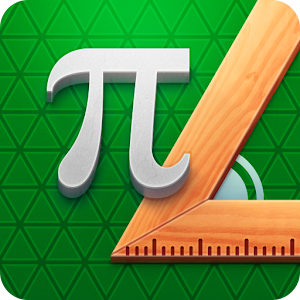# Pythagorea 60Â° - Lumos Educational App Store1
Price -
\$

#### DESCRIPTION:

Check how well you know the geometry by solving construction problems on a triangular grid. > 277 tasks: from very simple to really hard > 24 subjects to explore > 66 geometric terms in a glossary...

#### OVERVIEW:

Pythagorea 60Â° is a free educational mobile app By .It helps students in grades 8 practice the following standards 8.G.B.6,8.G.B.7,8.G.B.8.

This page not only allows students and teachers download Pythagorea 60Â° but also find engaging Sample Questions, Videos, Pins, Worksheets, Books related to the following topics.

1. 8.G.B.6 : Explain a proof of the Pythagorean Theorem and its converse. .

2. 8.G.B.7 : Apply the Pythagorean Theorem to determine unknown side lengths in right triangles in real-world and mathematical problems in two and three dimensions..

3. 8.G.B.8 : Apply the Pythagorean Theorem to find the distance between two points in a coordinate system..

8

#### STANDARDS:

8.G.B.6
8.G.B.7
8.G.B.8
8.G.A.1.B
8.G.A.1.C
8.G.A.2
8.G.A.3
8.G.A.4
8.G.A.5

Developer:

Software Version:

Category:

### RELATED APPSEdSearch WebSearch